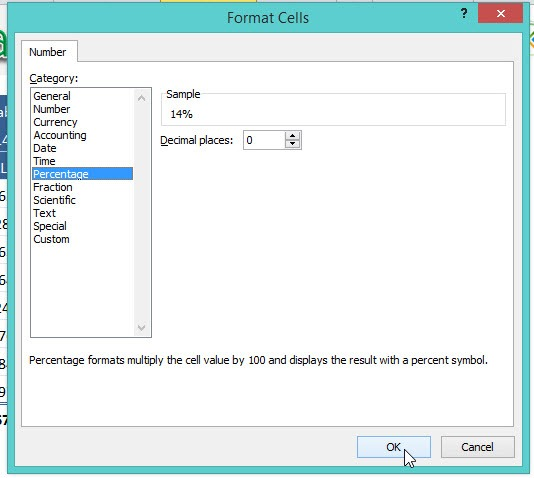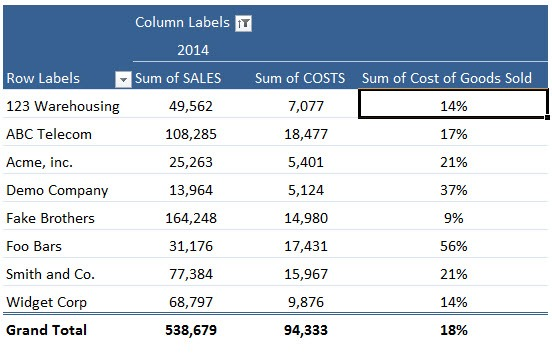Pivot Table Calculated Fields allow you to do mathematical calculations with your Field List.  You can use any of the Excel mathematical equations, like /*+-%.

The only limitation is that you cannot reference any cells.  Pivot Table Calculated Fields can be used to calculate percentage increases on Sales, margin calculations, or Cost of Goods sold, as I show below.

Here is our Pivot Table: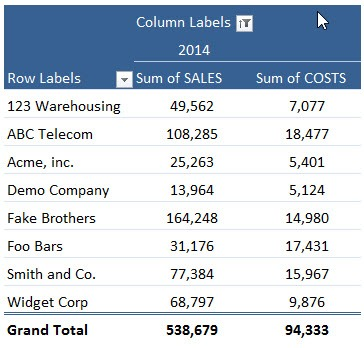STEP 1: Click on the Pivot Table and Go to Options > Fields, Items, & Sets > Calculated FieldSTEP 2: Set the Name to Cost of Goods Sold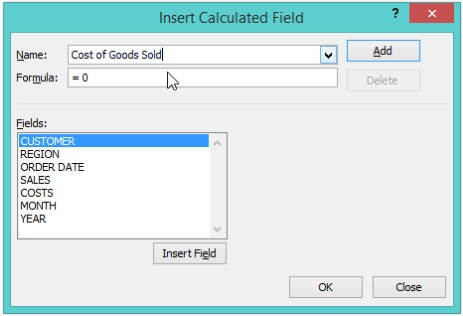STEP 3: Set the Formula to COSTS/SALES. This is the formula to be used for our calculated field.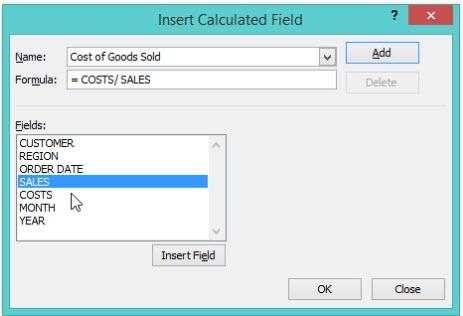STEP 4: The formatting is still not correct. Right click on the new column and select Number Format.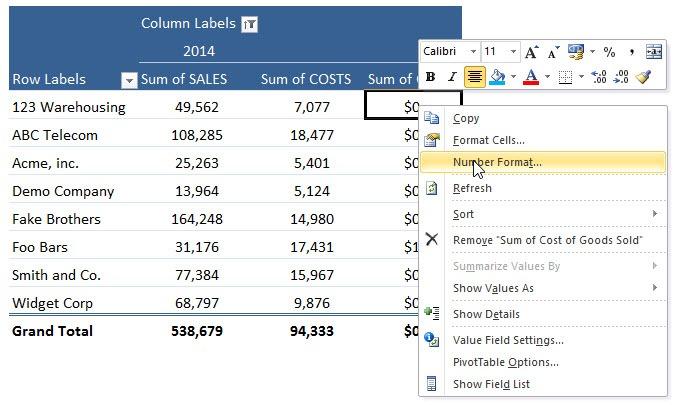STEP 5: Select Percentage and click OK.